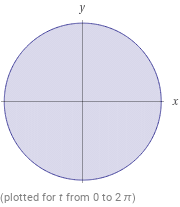Search IntMath
Close

450+ Math Lessons written by Math Professors and Teachers

5 Million+ Students Helped Each Year

1200+ Articles Written by Math Educators and Enthusiasts

Simplifying and Teaching Math for Over 23 Years

# Area of a Circle in Geometry## What is the Area of a Circle?

The area of a circle is the space enclosed by a closed curve. In geometry, the area of a circle is the amount of space occupied by the circle. It is measured in square units, such as cm� or m�. The area of a circle is calculated using the formula A = pr�, where A is the area, p is the ratio of a circle's circumference to its diameter, and r is the radius of the circle.

## What is the Circumference of a Circle?

The circumference of a circle is the distance around the edge of the circle. It is measured in linear units, such as cm or m. The circumference of a circle is calculated using the formula C = 2pr, where C is the circumference, p is the ratio of a circle's circumference to its diameter, and r is the radius of the circle.

## What is the Radius of a Circle?

The radius of a circle is the distance from the center of the circle to any point on the edge of the circle. It is measured in linear units, such as cm or m. The radius of a circle can be calculated using the formula r = C/2p, where C is the circumference and p is the ratio of a circle's circumference to its diameter.

## How to Calculate the Area of a Circle?

To calculate the area of a circle, you must use the formula A = pr�. To use the formula, you need to know the circle's radius. You can find the radius by measuring the distance from the center of the circle to any point on the edge of the circle. Once you have the radius, you can calculate the area of the circle by multiplying the radius by itself and then multiplying the result by the constant pi (p).

## Example Problems

Let's try a few example problems to practice calculating the area of a circle.

• 1. Find the area of a circle with a radius of 5 cm.
• A = pr� = 3.14 x 5� = 78.5 cm�
• 2. Find the area of a circle with a circumference of 25 cm.
• A = pr� = 3.14 x (25/2p)� = 98.5 cm�
• 3. Find the area of a circle with a diameter of 8 cm.
• A = pr� = 3.14 x (8/2)� = 50.24 cm�
• 4. Find the area of a circle with a radius of 7 m.
• A = pr� = 3.14 x 7� = 153.86 m�
• 5. Find the area of a circle with a circumference of 15 m.
• A = pr� = 3.14 x (15/2p)� = 88.36 m�
• 6. Find the area of a circle with a diameter of 10 m.
• A = pr� = 3.14 x (10/2)� = 78.5 m�

## Summary

In this lesson, we learned about the area of a circle in geometry. We discussed what the area of a circle is, what the circumference of a circle is, and what the radius of a circle is. We also learned how to calculate the area of a circle using the formula A = pr�. Finally, we practiced calculating the area of a circle with a few example problems.

## FAQ

### What is the formula to calculate the area of a circle?

The formula to calculate the area of a circle is A = pr2, where A is the area and r is the radius of the circle.

### What is the unit of measure for area of a circle?

The unit of measure for area of a circle is usually square units, such as square centimeters, square meters, etc.

## Problem SolverThis tool combines the power of mathematical computation engine that excels at solving mathematical formulas with the power of GPT large language models to parse and generate natural language. This creates math problem solver thats more accurate than ChatGPT, more flexible than a calculator, and faster answers than a human tutor. Learn More.